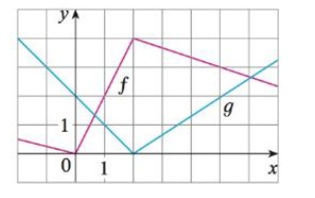Chapter 3.2, Problem 49E### Single Variable Calculus: Early Tr...

8th Edition
James Stewart
ISBN: 9781305270343

#### Solutions

Chapter
Section### Single Variable Calculus: Early Tr...

8th Edition
James Stewart
ISBN: 9781305270343
Textbook Problem

# If f and g are the functions whose graphs are shown, let u(x) = f(x)g(x) and v(x) = f(x)/g(x).(a) Find u'(l).(b) Find v'(5).(a)

To determine

To find: The derivative of u(x) at x=1.

Explanation

Given:

The function is u(x)=f(x)g(x).

Derivative rule:

Product Rule: ddx[f1(x)f2(x)]=f1(x)ddx[f2(x)]+f2(x)ddx[f1(x)]

Calculation:

Obtain the value of u(1).

u(x)=ddx(f(x)g(x))

Apply the product rule (1) and simplify the terms,

u(x)=f(x)ddx(g(x))+g(x)ddx(f(x))=f(x)g(x)+g(x)f(x)

Substitute 1 for x in u(x),

u(1)=f(1)g(1)+g(1)f(1) (1)

From the given graph, it is observed that f(1)=2 , g(1)=1 , the function f(x) is linear from (0,0) to (2,4) and the function g(x) is linear that passes through the points (0,2) and (2,0)

(b)

To determine

To find: The derivative of v(x) at x=5.

### Still sussing out bartleby?

Check out a sample textbook solution.

See a sample solution

#### The Solution to Your Study Problems

Bartleby provides explanations to thousands of textbook problems written by our experts, many with advanced degrees!

Get Started

#### Find more solutions based on key concepts